UPSC  >  NCERT Solutions: Structure of the Atom

# Structure of the Atom NCERT Solutions - Science & Technology for UPSC CSE

 Table of contentsPage No. 39Page No. 41Page No. 42Page No. 44Page No. 45Page No. 46, 47 & 48## Page No. 39

Q1. What are canal rays?
Ans: Canal rays, discovered by Goldstein in 1886, are positively charged radiation found in a gas discharge, which played a significant role in the discovery of protons, a subatomic particle.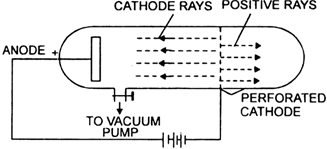Goldstein's experimental setup
Q2. If an atom contains one electron and one proton, will it carry any charge or not?
Ans: No, it will not carry any charge because positively charged particles are equal to negatively charged particles.

## Page No. 41

Q1. On the basis of Thomson's model of an atom, explain how the atom is neutral as a whole.
Ans: According to Thomson’s model, an atom consists of a sphere of positive charge and the electrons are embedded into it in such a way to give the most stable electrostatic arrangement.
This model explains the neutrality of the atom because an equal number of electrons and positively charged particles are embedded together.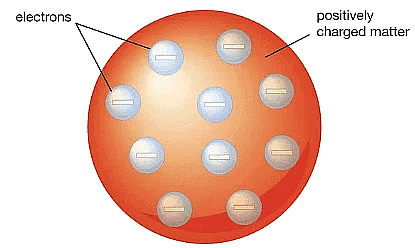Thomson's Model of an atom

Q2. On the basis of Rutherford's model of an atom, which subatomic particle is present in the nucleus of an atom?
Ans: On the basis of Rutherford's model, protons are present in the nucleus of an atom because the nucleus is positively charged. Since the whole mass of the atom is concentrated in the centre called the nucleus, therefore, neutrons are also present in the nucleus.Rutherford's Model of an atom
Q3. Draw a sketch of Bohr's model of an atom with three shells.
Ans: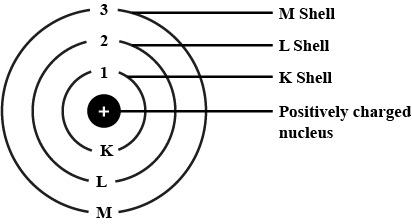A line diagram showing different energy levels of energies E1, E2 and E3 for the electrons

Q4. What do you think would be the observation if the α-particle scattering experiment is carried out using a foil of a metal other than gold?
Ans: If the metal taken is heavier than gold then the deviation shown by α-particles will be more and if metal taken is lighter than gold, then the deviation shown will be less due to less repulsion between positively a-particles and nucleus.

Q5. Name the three sub-atomic particles of an atom.
Ans: The three sub-atomic particles of an atom are:
(i) Protons
(ii) Electrons
(iii) Neutrons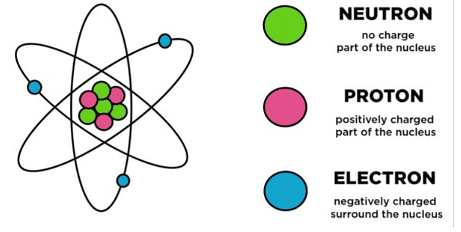Q6. Helium atom has an atomic mass of 4 u and two protons in its nucleus. How many neutrons does it have?
Ans: Number of neutrons = Atomic mass - Number of protons
Therefore, the number of neutrons in the atom = 4 - 2 = 2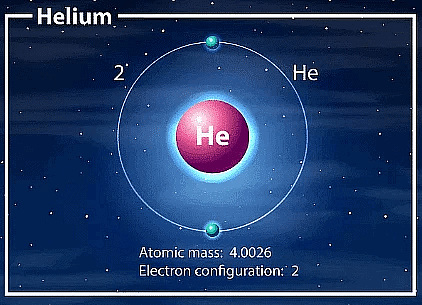## Page No. 42

Q1. Write the distribution of electrons in carbon and sodium atoms.
Ans:
(a) Electronic distribution in carbon atom
Atomic number of carbon = 6
Number of electrons = 6
Distribution of electrons: K = 2, L= 4
(b) Distribution of electrons in sodium atom
Atomic number of sodium atom =11
Number of electrons in sodium atom =11
Distribution of electrons in sodium atom: K = 2, T = 8, M = 1

Q2. If K and L shell of an atom are full, then what would be the total number of electrons in the atom?
Ans. The maximum capacity of K shell is 2 electrons and L shell can accommodate a maximum of 8 electrons in it. Therefore, there will be ten electrons in the atom.

## Page No. 44

Q1. How will you find the valency of chlorine, sulphur and magnesium?
Ans:

•  Cl (17) has 17 electrons. Its electronic configuration is 2, 8,-7. Tt can gain 1 electron to become stable like noble gas argon, so, its valency is equal to 1.
• S (16) has 16 electrons. Its electronic configuration is 2, 8, 6. It can gain 2 electrons to become stable like noble gas argon, so its valency is equal to 2.
• Mg (12) has 12 electrons. Its electronic configuration is 2, 8, 2. It can lose 2 electrons to become stable like noble gas neon, so, its valency is equal to 2.

Q2. If number of electrons in an atom is 8 and number of protons is also 8, then
(i) what is the atomic number of the atom and
(ii) what is the charge on the atom?
Ans:
(i) Atomic number = Number of protons = 8
(ii) Charge on atom is zero because the number of elections is equal to the number of protons.

Q3. With the help of Table 4.1, find out the mass number of oxygen and sulphur atom.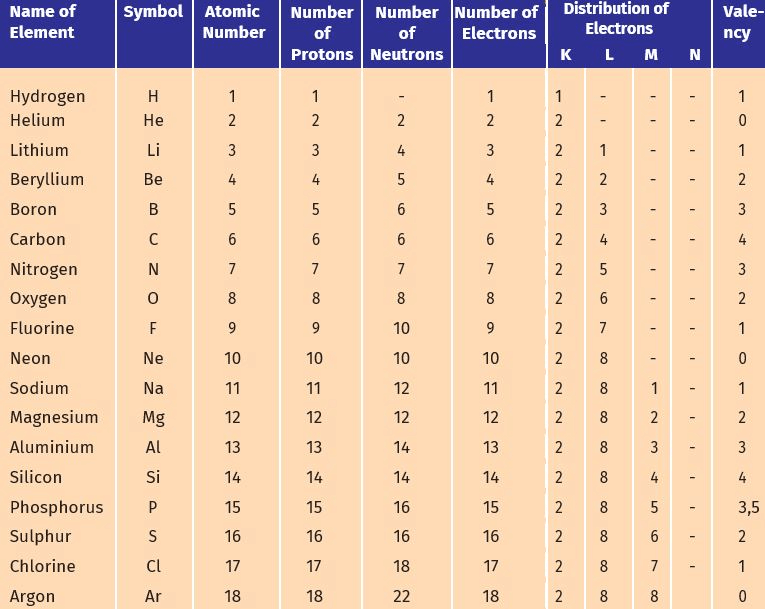Ans:
(a) To find the mass number of Oxygen:
Number of protons = 8
Number of neutrons = 8
Atomic number = 8
Atomic mass number = Number of protons + number of neutrons = 8 + 8 = 16
Therefore, mass number of oxygen = 16
(b) To find the mass number of Sulphur:
Number of protons = 16
Number of neutrons = 16
Atomic number = 16
Atomic mass number = Number of protons + number of neutrons = 16 + 16 = 32

## Page No. 45

Q1. For the symbol H, D and T tabulate three sub-atomic particles found in each of them.
Ans: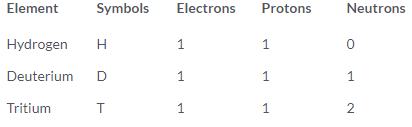Q2. Write the electronic configuration of any one pair of isotopes and isobars.
Ans:

• 12C6 and 14C6 are isotopes, have the same electronic configuration as (2, 4)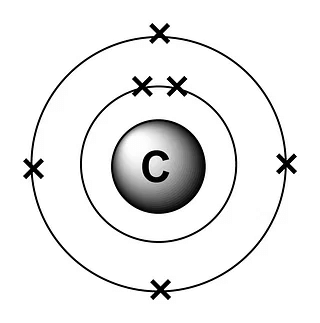Electronic Configuration(2,4) of Carbon atom

• 22Ne10 and 22Ne11 are isobars. They have different electronic configuration as given below:
22Ne10 – 2, 8
22Ne11 – 2, 8, 1

## Page No. 46, 47 & 48

Q1. Compare the properties of electrons, protons and neutrons.
Ans:

 Electron Proton Neutron (a) It is negatively charged. (a) It is positively charged. (a) It is neutral. (b) Its absolute mass is equal to 9.1 x 10-31 kg. (b) Its absolute mass is equal to 1.67 x 10-28 kg. (b) Its mass is slightly more than that of protons. Its absolute mass is 1.675 x 10-28 kg.

Q2. What are the limitations of J.J. Thomson's model of the atom?
Ans: Limitaions of JJ Thomson's model of an atom:
(i) The results of experiments carried out by other scientists could not be explained by this model
(ii) The positive charge is spread all over could not be justified.

Q3. What are the limitations of Rutherford's model of the atom?
Ans: Limitations of Rutherford's model of an atom
(i) According to classical electromagnetic theory, a moving charged particle, such as an electron under the influence of attractive force loses energy continuously in the form of radiations. As a result of this, the electron should lose energy and therefore, should move in even smaller orbits ultimately falling into the nucleus. But the collapse does not occur. There is no explanation for this behaviour.
(ii) According to Rutherford, the electrons revolve around the nucleus in fixed orbits. However, Rutherford did not specify the number of orbits and the number of electrons in each orbit.

Q4. Describe Bohr's model of the atom.
Ans: Bohr made a bold new suggestion that particles at the atomic level would behave differently from macroscopic (bigger) objects.
According to Bohr’s theory:
(i) Electrons revolve in certain orbits having well-defined energy.
(ii) Electrons do not emit radiations as long as they remain at the same energy level.
(iii) Supply of energy excites the electron to a higher energy level.
(iv) Emission of energy takes place when it jumps from higher energy level to lower energy level.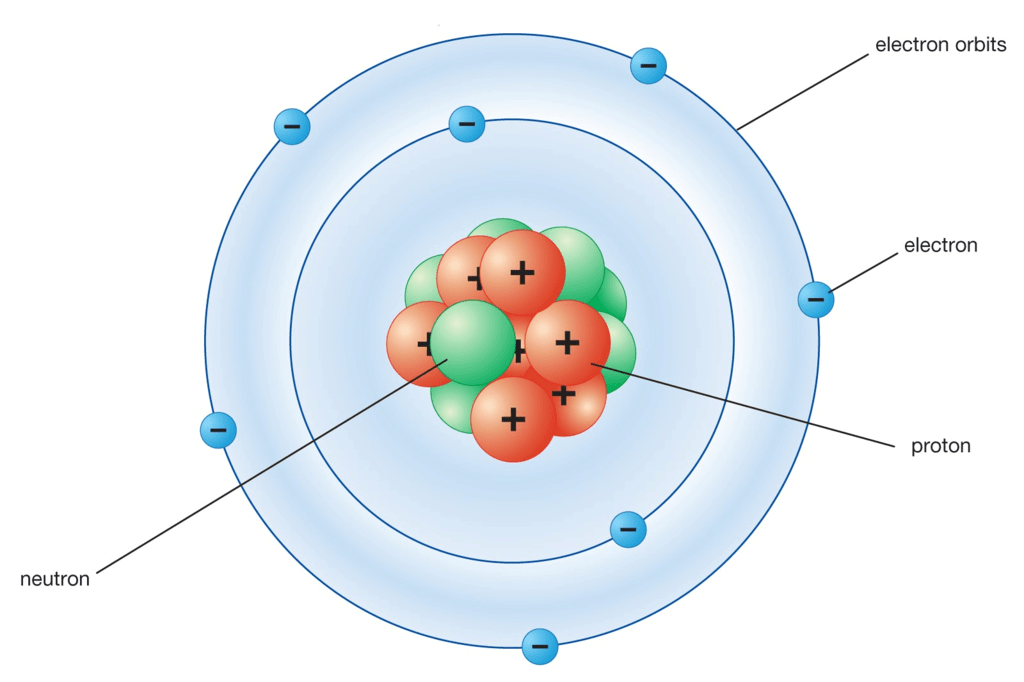Bohr's Model of an atom

Q5. Compare all the proposed models of an atom given in this chapter.
Ans:

 Thomson model Rutherford model Bohr model (a) The positive charge is spread all over and electrons are embedded in it like seeds in the watermelon. (a) There is a positively charged centre called the nucleus where the mass of the atom is concentrated. (a) Electrons revolve in certain discrete orbits called energy levels. (b ) The positive and negative charges are equal, therefore the atom is neutral. (b) Electrons revolve around the nucleus in well-defined orbits. (b) While revolving in these orbits, electrons do not radiate energy. (c) The size of the nucleus is very small as compared to the size of an atom. (c) These orbits are represented by K, L, M, N or 1, 2, 3, 4.

Q6. Summarize the rules for writing of distribution of electrons in various shells for the first eighteen elements.
Ans: The distribution of electrons in various shells of an atom is called electronic configuration.
In order to write the electronic configuration of an element, one should proceed as follows:
(i) With the help of atomic number of the element, find out the total number of electrons present in atom.
(ii) Allot these electrons in the various shells one by one in the order of increasing energy according to 2n2 rule.
(iii) The shell having least energy should be filled first and then the other shells of increasing energies are filled.
(iv) Write the name and number of electrons in various shells, starting with the lowest. Each number of electrons is separated from the other shell by a comma. For example, sodium (atomic number 11) has 11 electrons in its atom. According to 2n2 rule, K shell should be filled with 2 electrons and then L shell will be filled with 8 electrons and in the end, one left electron will go to N shell.
Example: Sodium (atomic number 11)
Electronic configuration of Na : K = 2, L = 8, M = 1

Q7. Define valency by taking examples of silicon and oxygen.
Ans: The valency of an atom is the number of hydrogen atoms which combines with one atom of an element.
For example,

• Silicon (atomic number 14) has the following electronic distribution:
K = 2, L = 8, M = 4.
In the outermost shell, there are 4 electrons hence one atom of silicon will combine with 4 atoms of hydrogen. So, the valency of silicon is 4.
• In case of oxygen (atomic number 8), the electronic distribution in various shells is given below:
K = 2, L = 6
There are six valence electrons in the atom of oxygen. So, one atom of oxygen will combine with 2 atoms of hydrogen to make a noble gas configuration. Hence, the valency of oxygen is 2.

Q8. Explain with examples (i) Atomic number, (ii) Mass number, (iii) Isotopes and (iv) Isobars. Give any two uses of isotopes.
Ans:
(i) Atomic number: The atomic number of an atom is equal to the number of protons in the nucleus of an atom.
Atomic number ~ Number of protons.
(ii) Mass number: The total mass of the atom is due to the sum of the masses of protons and neutrons present in the nucleus. The mass number of an atom is equal to the sum of the number of protons and neutrons in the nucleus.
Mass number = Number of protons + Number of neutrons.
(iii) Isotopes: Isotopes are the atoms of the same element having the same atomic number but different mass number. For example, hydrogen has three isotopes. They are called protium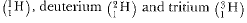(iv) Isobars: Isobars are the atoms of the different elements having the same mass number and different atomic number.
Uses of isotopes:
(i) Cobalt-60 is a radioactive isotope of cobalt. It is used in the radiotherapy of cancer.
(ii) 14C is a radioactive isotope of carbon. It is used in carbon dating.

Q9. Na+  has completely filled K and L shells. Explain.
Ans: Sodium has atomic number 11. It has 11 electrons in its orbitals wherein the number of protons is equal to the number of electrons. Hence, its electronic configuration is K-2; L-8; M-1; The one electron in the M shell is lost and it obtains a positive charge since it has one more proton than electrons, and obtains a positive charge, Na+. Na+ is formed when one electron is lost by a sodium atom as given below: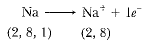The new electronic configuration is K-1; L-8 which is the filled state. Hence it is very difficult to eliminate the electron from a filled state as it is very stable.

Q10. If bromine atom is available in the form of, say, two isotopes  79Br35 (49.7%) and 81Br35 (50.3%) calculate the average atomic mass of bromine atom.
Ans: Average atomic mass of Br
= ( (79 x 49.7) + (81 x 50.3) ) / 100
= (3926.3 + 4074.3) / 100
= 8000.6 / 100
= 80.006 u

Q11. The average atomic mass of a sample of an element X is 16.2 u. What are the percentages of isotopes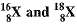in the sample?
Ans: It is given that the average atomic mass of the sample of element X is 16.2 u.
Let the percentage of isotope 18 / 8 X be y%. Thus, the percentage of isotope 16 / 8 X will be (100 - y) %.
Therefore,
18 x (y/100) + 16 x ((100-y)/100) = 16.2
18y/100 + ( 16(100 - y) / 100 ) = 16.2
(18y+1600-16y )/100 = 16.2
18y + 1600 - 16y = 1620
2y + 1600 = 1620
2y = 1620 - 1600
y = 10
Therefore, the percentage of isotope 18 / 8 X is 10%.
And, the percentage of isotope 16 / 8 X is (100 - 10) % = 90%.

Q12. If Z = 3, what would be the valency of the element? Also, name the element.
Ans. Z = 3
Electronic configuration is 2, 1.
Valence electron is equal to 1.
Valency is also equal to 1.
The name of the element is lithium.

Q13. Composition of the nuclei of two atomic species X and Y are given as under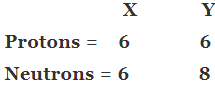Give the mass numbers of X and Y. What is the relation between the two species?
Ans: Mass number of X = 6 + 6 = 12
Mass number of Y = 6 + 8 = 14
Atomic number of X = 6
Atomic number of Y = 6
X and Y are isotopes since both the elements have same atomic number but different mass number.

Q14. For the following statements, write T for 'True' and F for 'False'.
(a) J.J. Thomson proposed that the nucleus of an atom contains only nucleons.

(b) A neutron is formed by an electron and a proton combining together. Therefore, it is neutral.
(c) The mass of an electron is about 1 / 2000 times that of proton.
(d) An isotope of iodine is used for making tincture iodine, which is used as a medicine.

Ans. (a) False (b) False (c) True (d) False

Q15. Rutherford's alpha-particle scattering experiment was responsible for the discovery of
(a) Atomic nucleus
(b) Electron
(c) Proton
(d) Neutron

Ans: (a) Atomic nucleus
An atomic nucleus is the dense central core of an atom, composed of protons and neutrons held together by the strong nuclear force.

Q16. Isotopes of an element have
(a) the same physical properties
(b) different chemical properties
(c) different number of neutrons
(d) different atomic numbers

Ans: (c) different number of neutrons

Q17. Number of valence electrons in Cl -ion are:
(a) 16
(b) 8
(c) 17
(d) 18

Ans: (b) 8
Cl- has 8 valence electrons. Cl- has 17 + 1 = 18 electrons. Its electronic configuration is 2, 8, 8.

Q18. Which one of the following is a correct electronic configuration of sodium?
(a) 2, 8
(b) 8, 2, 1
(c) 2, 1, 8
(d) 2, 8, 1

Ans: (d)  2 ,8 ,1
2 ,8 ,1 is the correct electronic configuration of sodium.

Q19. Complete the following table.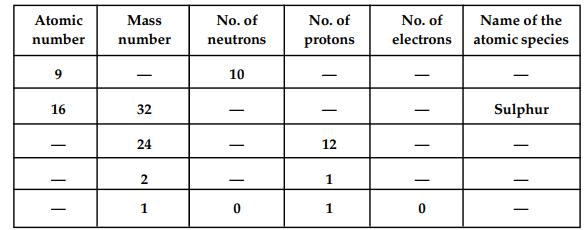Ans:
Atomic number(Z) =Number of protons
Mass number = Number of neutrons + atomic number
=> Mass number(A) = Number of neutrons + number of neutrons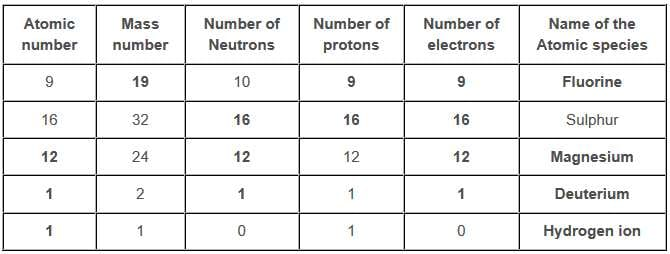The document Structure of the Atom NCERT Solutions | Science & Technology for UPSC CSE is a part of the UPSC Course Science & Technology for UPSC CSE.
All you need of UPSC at this link: UPSC

## Science & Technology for UPSC CSE

165 videos|449 docs|327 tests

## FAQs on Structure of the Atom NCERT Solutions - Science & Technology for UPSC CSE

 1. What is the structure of an atom?Ans. An atom consists of a positively charged nucleus composed of protons and neutrons, surrounded by negatively charged electrons in shells or energy levels.
 2. What is the difference between a proton and a neutron?Ans. A proton is a positively charged particle found in the nucleus of an atom, while a neutron is a neutral particle also found in the nucleus of an atom.
 3. How are electrons arranged in an atom?Ans. Electrons are arranged in shells or energy levels around the nucleus of an atom. The first shell can hold up to two electrons, while the second and third shells can hold up to eight electrons each.
 4. What is the atomic number of an element?Ans. The atomic number of an element is the number of protons in the nucleus of an atom of that element. It determines the chemical properties of the element.
 5. What are isotopes?Ans. Isotopes are atoms of the same element that have the same number of protons but a different number of neutrons in their nucleus. This results in isotopes having different atomic mass numbers.

## Science & Technology for UPSC CSE

165 videos|449 docs|327 testsExplore Courses for UPSC exam### How to Prepare for UPSC

Read our guide to prepare for UPSC which is created by Toppers & the best Teachers
Signup to see your scores go up within 7 days! Learn & Practice with 1000+ FREE Notes, Videos & Tests.
10M+ students study on EduRev
Track your progress, build streaks, highlight & save important lessons and more!
Related Searches

,

,

,

,

,

,

,

,

,

,

,

,

,

,

,

,

,

,

,

,

,

;